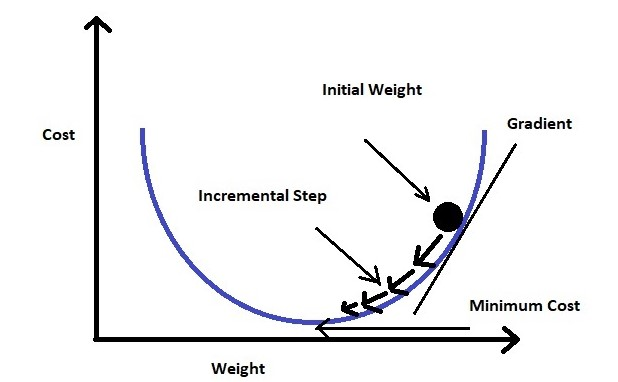# 逻辑回归讲解

import pandas as pd
import numpy as np
import matplotlib.pyplot as plt
import seaborn as sns
from collections import Counter
import random


## 数据介绍 #

file = '../static/files/large/mnist_train.csv'

df = pd.read_csv(file)

df.shape

(59999, 785)

df.head(2)


5 0 0.1 0.2 0.3 0.4 0.5 0.6 0.7 0.8 ... 0.608 0.609 0.610 0.611 0.612 0.613 0.614 0.615 0.616 0.617
0 0 0 0 0 0 0 0 0 0 0 ... 0 0 0 0 0 0 0 0 0 0
1 4 0 0 0 0 0 0 0 0 0 ... 0 0 0 0 0 0 0 0 0 0

2 rows × 785 columns

df.iloc[1,:].max()

255

def data_loader(file):
x = (df.iloc[:, 1:]/255.0).to_numpy()
y = df.iloc[:, 0].to_numpy()
return (x, y)

x_train, y_train = data_loader(file)

x_train

array([[0., 0., 0., ..., 0., 0., 0.],
[0., 0., 0., ..., 0., 0., 0.],
[0., 0., 0., ..., 0., 0., 0.],
...,
[0., 0., 0., ..., 0., 0., 0.],
[0., 0., 0., ..., 0., 0., 0.],
[0., 0., 0., ..., 0., 0., 0.]])

y_train

array([0, 4, 1, ..., 5, 6, 8])

example_to_draw = x_train[0, :].reshape(28,28)
plt.imshow(example_to_draw, cmap='gray')
plt.show()test_labels = [8, 4]
indices = np.where(np.isin(y_train, test_labels))

x = x_train[indices]
y = y_train[indices]

# 如果数字是 8， 我们把它变成 0
y[y == test_labels] = 0

# 如果数字是 4， 我们把它变成 1
y[y == test_labels] = 1


## 逻辑回归的原理 #

x.shape, y.shape

# 我们总共有 11693 个例子。x 的每一行是一个数字。

((11693, 784), (11693,))

example_to_draw = x[345, :].reshape(28,28)
plt.imshow(example_to_draw, cmap='gray')
plt.show()

# 这是一个 8# x[0, :]


m = x.shape

w = np.random.rand(m)
b = np.random.rand()

def check_random_pred_result(n):
"""随机抽 n 个在 0 和 len(x)+ 1 之间的整数，比较真实结果和基于 w, b 的预测结果
"""
rand_idxs = random.sample(range(0, len(x) + 1), n)
for i in rand_idxs:
h = x[i, :]@w + b
a = str(1/(1+np.exp(-h)))
result = str(y[i])
print("Actual result is "+ result + ", prediction is " + a)

check_random_pred_result(10)

Actual result is 0, prediction is 1.0
Actual result is 1, prediction is 0.9999999999999629
Actual result is 0, prediction is 0.9999999999999998
Actual result is 0, prediction is 0.9999999999999998
Actual result is 0, prediction is 1.0
Actual result is 0, prediction is 1.0
Actual result is 0, prediction is 1.0
Actual result is 1, prediction is 1.0
Actual result is 1, prediction is 1.0
Actual result is 1, prediction is 1.0

np.log(0.1)

-2.3025850929940455


$$L = - (y \cdot log(a) + (1-y)\cdot log(1-a))$$

$$L = - (0 \cdot log(1) - 1 \cdot log(0.001))$$

$$a = \frac{1}{1 + e^{-h}}$$

$$h = w^T \cdot x + b$$

$$\frac{\partial L}{\partial w} = \frac{\partial L}{\partial a} \cdot \frac{\partial a}{\partial h} \cdot \frac{\partial h}{\partial w}$$

$$\frac{\partial L}{\partial a} = \frac{a - y}{a (1-a)}$$

(如果上面这个你不知道是为啥，请看这里 )。

$$\frac{\partial a}{\partial h} = a(1-a)$$

(如果上面这个你不知道是为啥，请看这里 )。

$$\frac{\partial h}{\partial w} = x$$

$$\frac{\partial L}{\partial w} = x(a-y)$$$$\frac{\partial L}{\partial b} = \frac{\partial L}{\partial a} \cdot \frac{\partial a}{\partial h} \cdot \frac{\partial h}{\partial b}$$

$$\frac{\partial h}{\partial b} = 1$$

$$\frac{\partial L}{\partial b} = a-y$$

## 逻辑回归的代码实现 #

epochs = 20
alpha = 0.001
loss_prev = 10e6

w = np.random.rand(m)
b = np.random.rand()

for epoch in range(epochs):
# x: n * 784, w: 784 * 1, b: 1
# h: n * 1
a = x@w + b

# a: n * 1
a = 1/(1+np.exp(-a))

# 避免 log(0) 的情况
a = np.clip(a, .001, .999)

# y: n * 1, a - y: n * 1, x.T: 784 * n
# w: 784 * 1
w -= alpha * (x.T)@(a-y)

# 所有数据针对 b 的梯度下降取平均值
b -= alpha * np.mean(a - y)

loss = - np.sum(y*np.log(a) + (1-y)*np.log(1-a))
loss_reduction = loss_prev - loss
loss_prev = loss

accuracy = sum((a > 0.5).astype(int) == y)/len(y)

print('第', epoch+1, '轮', ' 损失为 {:.7}'.format(loss), \
' 该轮中损失减少了 {:.7}'.format(loss_reduction), \
' 正确识别率为 {:.4%}'.format(accuracy)
)

第 1 轮  损失为 40423.12  该轮中损失减少了 9.959577e+06  正确识别率为 49.9615%



## 检测 #

x_test, y_test = data_loader('../static/files/large/mnist_test.csv')
test_labels = [8, 4]
indices = np.where(np.isin(y_test, test_labels))

x = x_test[indices]
y = y_test[indices]

# 如果数字是 8， 我们把它变成 0
y[y == test_labels] = 0

# 如果数字是 4， 我们把它变成 1
y[y == test_labels] = 1

h = x@w + b
a = 1/(1+np.exp(-h))
accuracy = sum((a > 0.5).astype(int) == y)/len(y)
print(' 正确识别率为 {:.4%}'.format(accuracy))

 正确识别率为 98.7219%

#ML